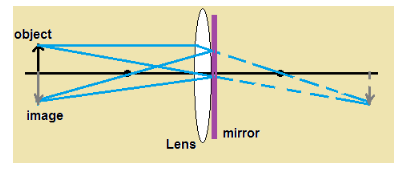# A convex lens is placed in contact with a plane mirror. a point object

A convex lens is placed in contact with a plane mirror. a point object is placed 20cm on the axis of the combination has its image coinciding with itself.what is the focal length of the lens?The figure shown above describes the problem. If we map the reflected ray in the other side of mirror as the image of mirror, then the ray diagram is same as for the object placed at 2F in front of convex lens. If the object is at 2F of one side of lens, then we get image at 2F of other side. Since object to lens distance is given as 20 cm in this problem, the focal length of the mirror is 10 cm